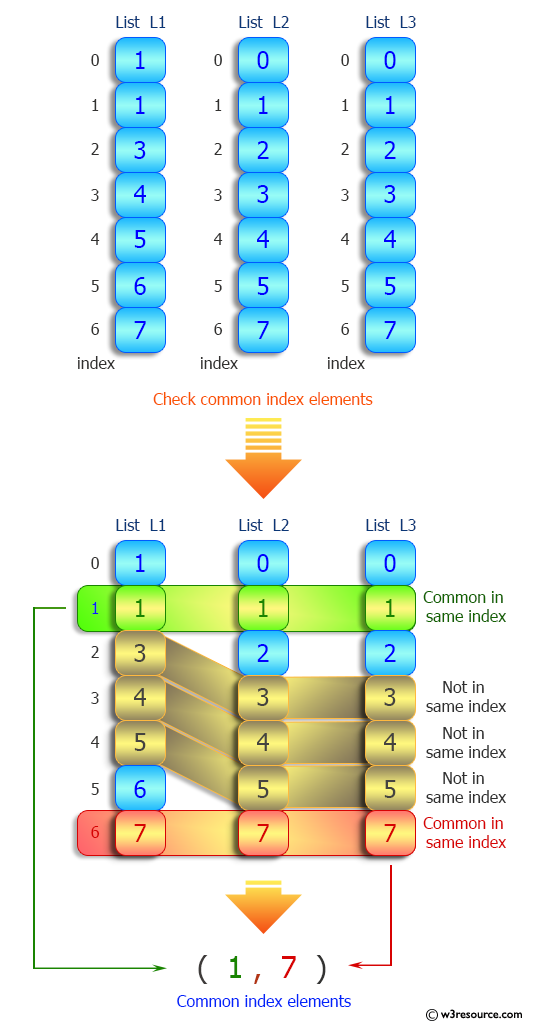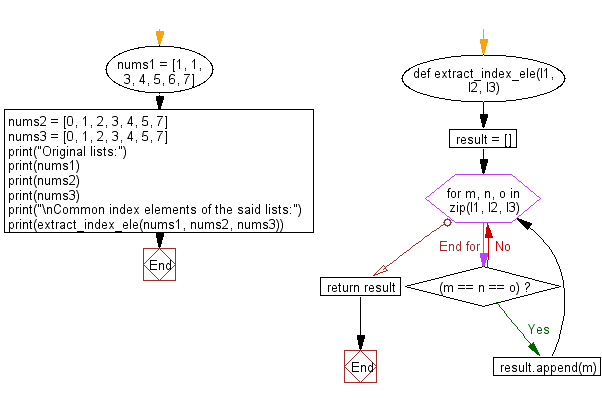﻿ Python: Common index elements from more than one list - w3resource# Python: Common index elements from more than one list

## Python List: Exercise - 100 with Solution

Write a Python program to extract common index elements from more than one given list.

Sample Solution:

Python Code:

``````def extract_index_ele(l1, l2, l3):
result = []
for m, n, o in zip(l1, l2, l3):
if (m == n == o):
result.append(m)
return result

nums1 = [1, 1, 3, 4, 5, 6, 7]
nums2 = [0, 1, 2, 3, 4, 5, 7]
nums3 = [0, 1, 2, 3, 4, 5, 7]

print("Original lists:")
print(nums1)
print(nums2)
print(nums3)
print("\nCommon index elements of the said lists:")
print(extract_index_ele(nums1, nums2, nums3))
```
```

Sample Output:

```Original lists:
[1, 1, 3, 4, 5, 6, 7]
[0, 1, 2, 3, 4, 5, 7]
[0, 1, 2, 3, 4, 5, 7]

Common index elements of the said lists:
[1, 7]
```

Pictorial Presentation:Flowchart:## Visualize Python code execution:

The following tool visualize what the computer is doing step-by-step as it executes the said program:

Python Code Editor:

Have another way to solve this solution? Contribute your code (and comments) through Disqus.

What is the difficulty level of this exercise?

Test your Python skills with w3resource's quiz

﻿

## Python: Tips of the Day

```print(2_000_000)
```2000000